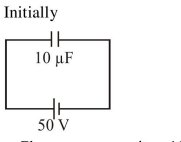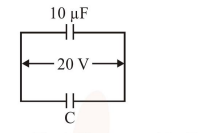# Solve this following

Question:

A $10 \mu \mathrm{F}$ capacitor is fully charged to a potential difference of $50 \mathrm{~V}$. After removing the source voltage it is connected to an uncharged capacitor in parallel. Now the potential difference across them becomes $20 \mathrm{~V}$. The capacitance of the second capacitor is:

1. $10 \mu \mathrm{F}$

2. $15 \mu \mathrm{F}$

3. $20 \mu \mathrm{F}$

4. $30 \mu \mathrm{F}$

Correct Option: 2,

Solution:- Charge on capacitor $10 \mu \mathrm{F}$

$\mathrm{Q}=\mathrm{CV}=(10 \mu \mathrm{F})(50 \mathrm{~V})$

$\mathrm{Q}=500 \mu \mathrm{C}$- Final Charge on $10 \mu \mathrm{F}$ capacitor

$\mathrm{Q}=\mathrm{CV}=(10 \mu \mathrm{F})(20 \mathrm{~V})$

$\mathrm{Q}=200 \mu \mathrm{C}$

- From charge conservation,

Charge on unknown capacitor

$C=500 \mu C-200 \mu C=300 \mu C$

$\Rightarrow$ Capacitance $(\mathrm{C})=\frac{\mathrm{Q}}{\mathrm{V}}=\frac{300 \mu \mathrm{C}}{20 \mathrm{~V}}=15 \mu \mathrm{F}$